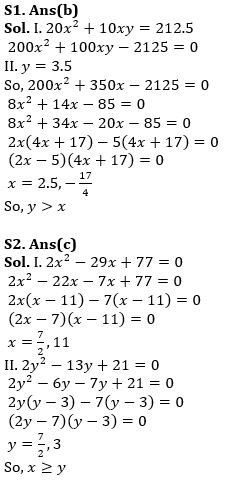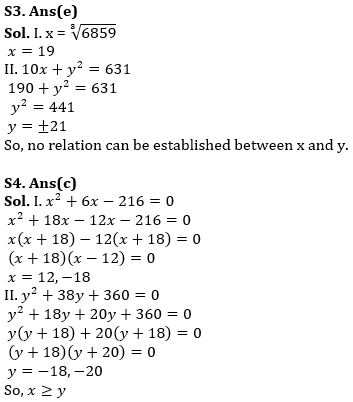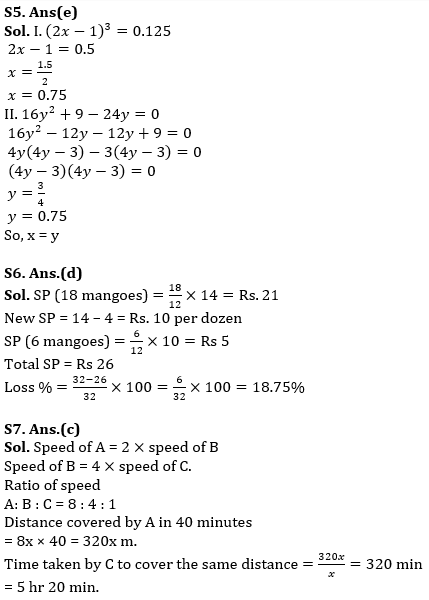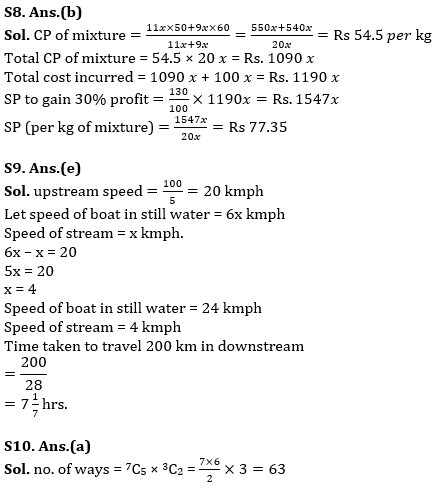Latest Banking jobs   »   Quantitative Aptitude Quiz For RBI Assistant/...

# Quantitative Aptitude Quiz For RBI Assistant/ ESIC UDC Mains 2022- 17th April

Directions (1-5): In each of the following questions two equations (I) and (II) are given, you have to solve both the equation and give answer
(a) if x > y
(b) if x < y
(c) if x ≥ y
(d) if x ≤ y
(e) if x = y or no relationship can be obtained between x and y.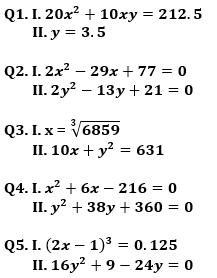Q6. The CP of two dozen mangoes is Rs 32, after selling 18 mangoes at Rs 14 per dozen, the shopkeeper reduced the price by Rs 4 per dozen. Find his loss percentage.
(a) 15%
(b) 18%
(c) 20 %
(d) 18.75%
(e) 22%

Q7. A is twice as fast as B while B is 4 times as fast as C. If A covers a certain distance in 40 minutes, then find the time taken by C to cover the same distance.
(a) 5 hr
(b) 1 hr 20 min
(c) 5 hr 20 min
(d) 4 hr 40 min
(e) 5 hr 30 min

Q8. A vendor mixes two types of rice costing Rs. 50 per kg and Rs. 60 per kg in ratio of 11 : 9. At what price (Rs. Per kg) should he sell the mixture to gain 30% profit, if he incurs Rs. 5/kg on transportation?
(a) 54.5
(b) 77.35
(c) 59.5
(d) 85
(e) 70

Q9. A person when rows upstream covers a distance of 100km in 5 hours. Find the time taken (in hrs) by the person to travel 200 km downstream if speed of boat in still water is 6 times the speed of stream.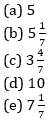Q10. In how many ways can a group of 5 men & 2 women be made out of 7 men & 3 women?
(a) 63
(b) 45
(c) 126
(d) 90
(e) 84

Solutions